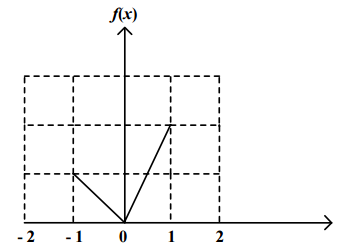### CAT 2000 Question Paper Question 121

Instructions

Directions for the next 3 questions:

Given below are three graphs made up of straight-line segments shown as thick lines. In each case choose the answer as:

a) if f(x)=3f(-x)

b) if f(x)= -f(-x)

c) if f(x) = f(-x)

d) if 3f(x) = 6f(-x), for x >= 0

Question 121

#Solution

In this graph, f(-1) = 1 and f(1) = 2 and since the two lines pass origin, their values increase linearly.

3f(x) = 6f(-x)